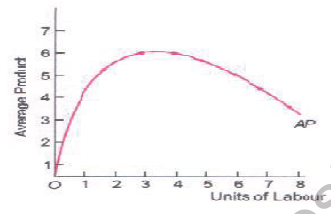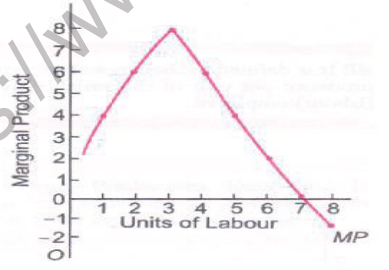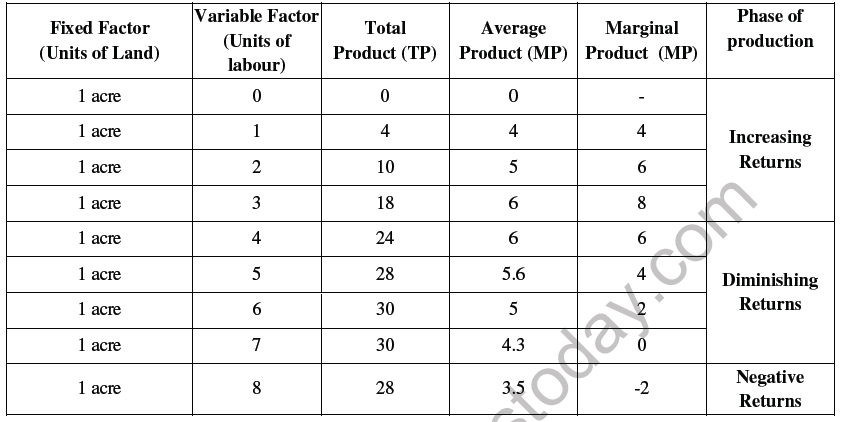# CBSE Class 11 Economics Production Function Worksheet

Download printable Economics Class 11 Worksheets in pdf format, CBSE Class 11 Economics Production Function Worksheet has been prepared as per the latest syllabus and exam pattern issued by CBSE, NCERT and KVS. Also download free pdf Economics Class 11 Assignments and practice them daily to get better marks in tests and exams for Grade 11. Free chapter wise worksheets with answers have been designed by Standard 11 teachers as per latest examination pattern

INTRODUCTORY MICROECONOMICS

PRODUCTION FUNCTION ( RETURNS TO FACTOR)

MEANING OF PRODUCTION

Production is defined as the transformation of inputs into output. Production includes both production of physical goods and production of services

→ PRODUCTION FUNCTION

• The term production function means the technical and physical relationship between inputs used and the resulting output.

• It includes only technically efficient combinations of inputs (i.e., those which minimise the cost of production).

• A production function is expressed as:
Q=f (K, L) Where Q is output, labour (L) and capital (K), required to produce a good
Types of Production Function

• Short-run Production Function. It refers to production in the short-run where there are some fixed factors. In short-run, production increases when more units of variable factors are used with certain amount of fixed factor.

• Long-run Production Function. It refers to production when all factors are increased in the same proportion Fixed Factors and Variable Factors Fixed factors refer to those factors whose supply cannot be changed during short run. E.g. land, plant, factory building, minimum electricity bill, etc. Variable factors refer to those factors whose supply can be varied or changed. E.g. raw materials, daily wage workers, etc.

→ CONCEPTS OF PRODUCT

• Total Physical Product (TPP) or Total Product (TP): It is defined as the total quantity of goods and services produced by a firm with the given inputs during a specified period of time.

• In the short-run, TP can be increased by employing more units of the variable factor.

• In the long-run, TP can be increased by employing more units of all factors.

TP Curve

TP curve starts from the origin, increases at an increasing rate, reaches a maximum and finally decreases at an increasing rate→ Average Product (AP)

Average Product (AP): It is defined as the amount of output produced per unit of the variable factor (labour) employed

AP = Total physical product / Labour Input = TP / L

AP is zero when no labour is employed. Initially, AP increases, reaches maximum and finally starts declining. AP curve is an inverted U-shaped.→ Marginal Product (MP)

It is defined as the change in TP resulting from the employment of an additional unit of a variable factor (labour). symbolically, MP can be written as :Initially MP increases with employment of units of labour. MP starts declining to become zero and finally becomes negative. MP curve is inverted U-shaped.Relationship between TP, AP and MP Curves

• AP curve is the slope of the straight line from the origin to each point on the TP curve. MP curve is the slope of the TP curve at each point.

• When AP is maximum, MP = AP.

• When TP is maximum, MP = O.

• When TP is falling, MP is negative.

• Both AP and MP curves are inverted U-shaped.Law of Diminishing Marginal Product

If we keep increasing the employment of the variable input with other fixed inputs then eventually a point will be reached after which the marginal product of that input will start falling. MP of a factor input initially rises, when the level of employment of the input is low, but after reaching a certain level of employment, it starts falling.

The Law of Variable Proportion

Statement of the laws Law of Variable Proportion
The law of variable proportion states that when total output or production of a commodity is increased by adding units of a variable input, while the quantities of other inputs are held constant, the increase in total production, after some point, diminishes.

• Assumptions of the Law

• The assumptions of the law of variable proportion are:

• State of technology remains the same.

• All units of the variable factor, labour, are homogenous.

• There must always be some fixed input and diminishing returns results due to fixed supply of the fixed factor.

## Tags:

Click for more Economics Study Material
 CBSE Class 11 Economics Market Equilibrium Worksheet CBSE Class 11 Economics Worksheet Set H Solved CBSE Class 11 Economics Organisation of Data Worksheet Set D CBSE Class 11 Economics Index Number Worksheet CBSE Class 11 Economics Worksheet Set B Solved CBSE Class 11 Economics On Presentation of Data Worksheet CBSE Class 11 Micro Economics Indifference Curve Analysis Worksheet CBSE Class 11 Economics Collection of Data Worksheet Set B CBSE Class 11 Economics Statics For Economics Worksheet Set C CBSE Class 11 Economics Median And Quartiles Worksheet CBSE Class 11 Economics Worksheet Set I Solved CBSE Class 11 Economics Presentation of data Worksheet CBSE Class 11 Economics Indian Economy On The Eve Of Independence Worksheet CBSE Class 11 Economics Worksheet Set C Solved CBSE Class 11 Economics On Range and Quartile Deviation Worksheet CBSE Class 11 Micro Economics Introduction Worksheet CBSE Class 11 Economics Collection of Data Worksheet Set C CBSE Class 11 Economics Statics Introduction Worksheet CBSE Class 11 Economics Non Competitive Market Worksheet CBSE Class 11 Economics Worksheet Set J Solved CBSE Class 11 Economics Production And Cost Worksheet CBSE Class 11 Economics Indian Economy Worksheet CBSE Class 11 Economics Worksheet Set D Solved CBSE Class 11 Economics On Rural Development Worksheet CBSE Class 11 Micro Economics Price Elasticity of Demand Worksheet CBSE Class 11 Economics Comparative Development Experience Worksheet CBSE Class 11 Economics Statistics For Economics Worksheet CBSE Class 11 Economics On Employment Worksheet CBSE Class 11 Economics Worksheet Set K Solved CBSE Class 11 Economics Production Function Worksheet CBSE Class 11 Economics Introduction to Microeconomics Worksheet CBSE Class 11 Economics Worksheet Set E Solved CBSE Class 11 Economics Organisation of Data Worksheet Set A CBSE Class 11 Micro Economics Theory of Demand Worksheet CBSE Class 11 Economics Demand Analysis Worksheet Set A CBSE Class 11 Economics Theory of Firm Under Perfect Competition Worksheet CBSE Class 11 Economics On Human Capital Formation Worksheet CBSE Class 11 Economics Worksheet Set L Solved CBSE Class 11 Economics Production Possibility Curve Worksheet CBSE Class 11 Economics Introduction Worksheet Set A CBSE Class 11 Economics Worksheet Set F Solved CBSE Class 11 Economics Organisation of Data Worksheet Set B CBSE Class 11 Micro Economics Consumer Behaviour And Demand Utility Analysis Worksheet CBSE Class 11 Economics Elasticity of Demand Worksheet CBSE Class 11 Economics Utility Analysis Worksheet CBSE Class 11 Economics On Median And Quartiles Worksheet CBSE Class 11 Micro Economics Consumer Behaviour And Demand Analysis Worksheet CBSE Class 11 Economics Arithmetic Mean And Weighted Mean Worksheet CBSE Class 11 Economics Statics For Economics Worksheet Set A CBSE Class 11 Economics Introduction Worksheet CBSE Class 11 Economics Worksheet Set G Solved CBSE Class 11 Economics Organisation of Data Worksheet Set C CBSE Class 11 Economics Indefference Curve Analysis Worksheet CBSE Class 11 Economics Worksheet Set A Solved CBSE Class 11 Economics On Poverty Worksheet CBSE Class 11 Micro Economics Consumer Behaviour And Demand Indifference Analysis Worksheet Set A CBSE Class 11 Economics Collection of Data Worksheet Set A CBSE Class 11 Economics Statics For Economics Worksheet Set B

## Latest NCERT & CBSE News

Read the latest news and announcements from NCERT and CBSE below. Important updates relating to your studies which will help you to keep yourself updated with latest happenings in school level education. Keep yourself updated with all latest news and also read articles from teachers which will help you to improve your studies, increase motivation level and promote faster learning

### CBSE Board Examination Scheme of Assessment 2021 2022

A. Academic session to be divided into 2 Terms with approximately 50% syllabus in each term: The syllabus for the Academic session 2021-22 will be divided into 2 terms by following a systematic approach by looking into the interconnectivity of concepts and topics by...

### MCQ Question based CBSE examination

For 2021-22 CBSE has launched MCQ question-based examination for Term 1 & Term 2 board examinations. The entire syllabus has been divided into two parts each including 50% of the entire syllabus. To score well, students must practice as per the new CBSE term-wise...

### How To Solve Unseen Passages In English

Unseen passages may contain one or many paragraphs. This is one of the important yet easy parts for a student to get marks. Students should thoroughly study and understand the passage to answer the related questions. The unseen passages are there just to test the...

### CBSE Class 10 Revised Syllabus

Last year CBSE had to reduce the syllabus because of the pandemic situation but it was not very effective because there were no examinations. This year to avoid any confusion and conflict, CBSE has decided to reduce the syllabus into term 1 and term 2. 50 percent of...

### Score well in Class 12 English Boards Exam

12th Board exams are an important part of students' lives. The marks obtained in the board exam decide the college in which one can study. In class 12 the syllabus of each and every subject increases vastly and it is difficult to cover up every point. In English also...

### Moderation of Marks Class 11 and 12 Board Exams

The portal for moderation and finalization of results for Class-12 is being opened from 16.07.2021 to 22.07.2021. As Board has to declare the result latest by 31.07.2021, schools have been requested to follow the schedule strictly and complete the moderation within...

×